Courses

# Polarization - EM Waves, Electromagnetic Theory, CSIR-NET Physical Sciences Physics Notes | EduRev

## Physics for IIT JAM, UGC - NET, CSIR NET

Created by: Akhilesh Thakur

## Physics : Polarization - EM Waves, Electromagnetic Theory, CSIR-NET Physical Sciences Physics Notes | EduRev

The document Polarization - EM Waves, Electromagnetic Theory, CSIR-NET Physical Sciences Physics Notes | EduRev is a part of the Physics Course Physics for IIT JAM, UGC - NET, CSIR NET.
All you need of Physics at this link: Physics

In classical physics light is assumed to be an electromagnetic wave.  Electromagnetic waves are categorized according to their frequency f or, equivalently, according to their wavelength l.  The speed of any electromagnetic waves in free space is the speed of light c = 3*10m/s.  Electromagnetic waves can have any wavelength l or frequency f as long as lf = c.  Visible light has a wavelength range from ~400 nm to ~700 nm.  Violet light has a wavelength of ~400 nm, and a frequency of ~7.5*1014 Hz.  Red light has a wavelength of ~700 nm, and a frequency of ~4.3*1014 Hz.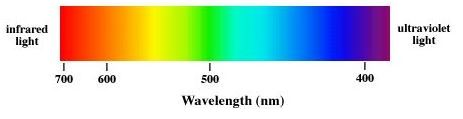Electromagnetic (EM) waves are changing electric and magnetic fields, carrying energy through space.  EM waves require no medium, they can travel through empty space.  Let E denote the electric field vector and B the magnetic field vector of the EM wave.  For electromagnetic waves E and B are always perpendicular to each other, and perpendicular to the direction of propagation of the wave.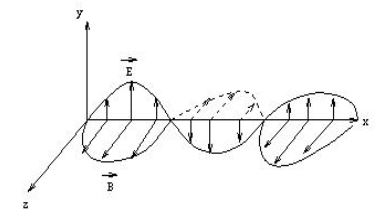Electromagnetic waves are transverse waves.

In general we pay more attention to the electric field E, because detectors such as the eye, photographic film, and CCDs interact with the electric field.

Polarization is a phenomenon peculiar to transverse waves.  Longitudinal waves such as sound cannot be polarized.  In the diagram below an EM wave is propagating in the x-direction, the electric field oscillates in the xy plane, and the magnetic field oscillates in the xz plane.  A line traces out the electric field vector as the wave propagates.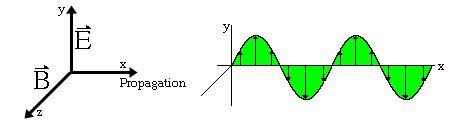An unpolarized electromagnetic wave traveling in the x-direction is a superposition of many waves.  For each of these waves the electric field vector is perpendicular to the x-axis, but the angle it makes with the y-axis is different for different waves.  For a polarized electromagnetic wave traveling in the x-direction, the angle the electric field makes with the y-axis is unique.  Natural light is, in general, unpolarized.  The direction of the electric field changes too quickly to be measured.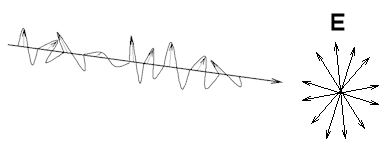A polarizer is a material that passes only EM waves for which the electric field vector is confined to a single plane that contains the direction of motion.   A polarizer has a transmission axis, and it passes the components (projections) of the electric field vectors that are parallel to its transmission axis.  If E0 is the incident field vector and the angle between E0 and the transmission axis is q, then the magnitude of transmitted field vector is E0cosq and its direction is the direction of the transmission axis.  The intensity I of an electromagnetic wave is proportional to the square of the magnitude of the electric field vector. We therefore have

Itransmitted = I0cos2q.

If q = 90o the transmitted intensity is zero.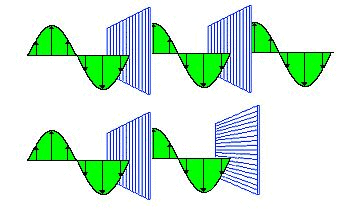A polarizer produces linearly polarized light.  It is often convenient to orient the transmission axis of a polarizer vertically or horizontally to produce light with vertical or horizontal linear polarization.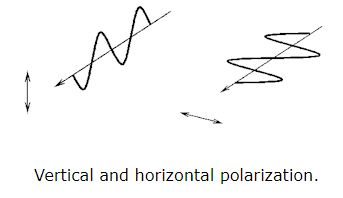• Polarizers

Light can also be elliptically or circularly polarized.  For light with circular polarization the electric field vector rotates in a plane perpendicular to the direction of propagation.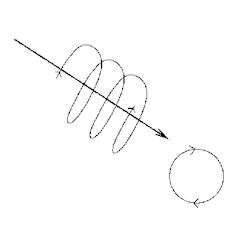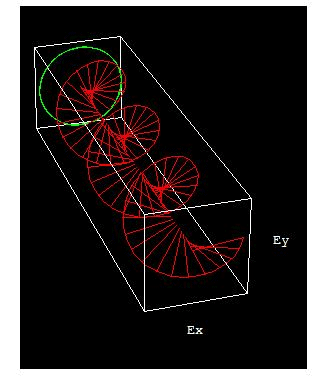Circularly polarized light propagating along the z-direction

• Polarized light waveforms

Polarization Mechanisms

Dichroism:  Certain naturally occurring crystalline materials have transmittance properties which depend on the polarization state of light.  The most common method of producing polarized light is to use polaroid material, made from chains of organic molecules, which are anisotropic in shape.  Light transmitted is linearly polarized perpendicular to the direction of the chains.  If linearly polarized light passes through polaroid material, then the transmitted intensity is given by I= I0cos2q, (Law of Malus), where q is the angle between E and transmission direction.

Reflection:  When unpolarized light is incident on a boundary between two dielectric surfaces, for example on an air-glass or air water boundary, then the reflected and transmitted components are partially polarized.  The reflected wave is 100% linearly polarized perpendicular to the plane of incidence when the incident angle is equal to the Brewster angle qB, where tanqB = n2/n1.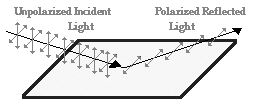When the sun is at a low angle in the sky, the sunlight reflecting off the surface of water is nearly 100% horizontally polarized because the angle of incidence is close to the Brewster angle.  Glare-reducing sunglasses are coated with a polarizer with a vertical transmission axis and therefore block the reflected light.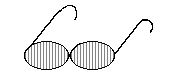• Brewster angle

Birefringence (double refraction):  Certain crystalline substances have a refractive index which depends upon the state of incident polarization.  Unpolarized light entering a birefringent crystal not along the optic axis of the crystal is split into two beams which are refracted by different amounts and polarized perpendicular to each other.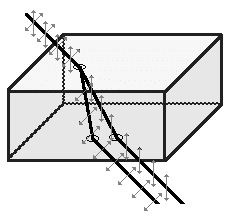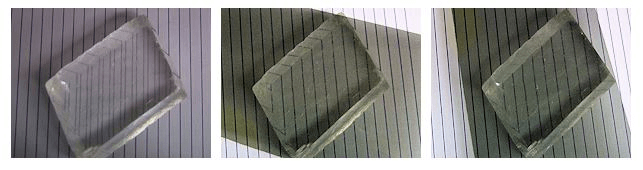Double refraction in a calcite crystal.  The vertical lines are imaged twice.
The polarization of the light producing one image is perpendicular to the
polarization of the light producing the other image.

We can cut birefringent crystals into quarter-wave plates, which can be used to convert linearly polarized light into circularly polarized light and vice versa.  Two quarter-wave plates in succession can turn vertically polarized light into horizontally polarized light and vice versa.

Optically active (circular birefringent) materials rotate the direction of polarization of linearly polarized light.  The amount of rotation depends on the wavelength of the light.  Sugar molecules have a handedness (chirality) and in solution are optically active.  If we polarize white light and pass it through sugar syrup, the direction of polarization of the light emerging from the syrup will be different for the different color components.  If the light then passes through a second polarizer, its color changes with the orientation of the transmission axis of this polarizer.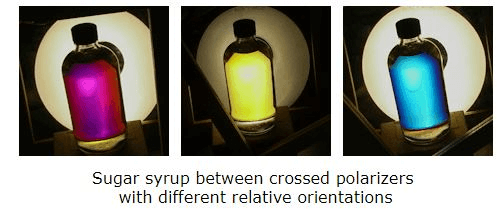Some materials turn birefringent when stressed.  By placing transparent materials between two polarizers, we can perform stress analysis tests.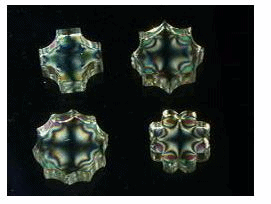Stressed glass between crossed polarizers

159 docs

,

,

,

,

,

,

,

,

,

,

,

,

,

,

,

,

,

,

,

,

,

,

,

,

,

,

,

;